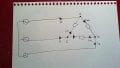# Three phase Delta connection with an unbalanced load

#### bosko47

Joined Mar 24, 2016
23I'm trying to get my head around what's happening in this diagram - A Delta connection with an unbalanced load.

For a balanced load, using KCL, the currents would be:
ia = iab - ica
ib = ibc - iab
ic = ica + icb

But what happens when there is an unbalanced load? I can't find a lot about unbalanced Delta loads in my textbooks or online...
Given these options, I can only find one that I think would be correct: ia + ib + ic = icirculating

ia = iac - iba
ic = iac + iab
iab = ia - ib
ibc = ic - iab
ia + ib + ic not equal to 0
ia + ib + ic = in
ia + ib + ic = icirculating
ib = iba - ibc
ib = iba - icb

*Note: I'm guessing that the unlabelled current in the diagram could be because of the unbalanced load(?) between point c and b

#### WBahn

Joined Mar 31, 2012
29,175
View attachment 149050

I'm trying to get my head around what's happening in this diagram - A Delta connection with an unbalanced load.

For a balanced load, using KCL, the currents would be:
ia = iab - ica
ib = ibc - iab
ic = ica + icb
Why would it be any different for an unbalanced load.

It's just a circuit that happens to have three sources. Analyze it as a circuit that has three sources.

#### Tech_Elect

Joined Feb 13, 2019
8
Hi,
it is an unbalanced load, so by definition the currents are not the same

you have to calculate and analyze each generator and each impedance to calculate the correct value of the currents flowing in an unbalanced load (consider even if the impedances are capacitive or inductive or resistive)

Regards